## Final Project Progress

#### Project Description:

My project is a generative drawing project where the user gets to draw with hands/joystick and then save the work they drew.

I started my work by exploring different shapes and types of brushes to choose one. After some time and looking around I decided it was best to give the user the option to use more than one brush in one piece.

I am still not done with the brush class but after testing the brushes separately, that is my unedited code for the brush class:

###### Main Class Function:
```import java.util.*;

class Brush {

int brushN;

float stepSize;
float angle;
float x, y, tempX, tempY;
color col;
//
int counter;
PFont font;
float fontSize;
String letters;
float  fontSizeMin;
//
float [] blurX={ -stepSize, -stepSize, 0, stepSize, stepSize, stepSize, 0, -stepSize};
float [] blurY={ 0, stepSize, stepSize, stepSize, 0, -stepSize, -stepSize, -stepSize};
//linemoduelw
PShape lineModule;

float  linelength=100;
float [] numX={ -100, -70, 0, 70, 100, 70, 0, -70};
float [] numY={ 0, 70, 100, 70, 0, -70, -100, -70};

Brush(int defaultt, float step_size) {
// 1 blur
// 2 random cylinder
// 3 dynamic shapes
// 4 text lines
// 5 rotating lines
brushN = defaultt;
stepSize = step_size;
angle = 270;
x = mouseX;
y = mouseY;
col = color(255);
fontSizeMin=200;

fontSize=50;
letters = "It isn't true that my mattress is made of cotton candy. It was the first time he had ever seen someone cook dinner on an elephant. Homesickness became contagious in the young campers' cabin. Every manager should be able to recite at least ten nursery rhymes backward.";
// for(int i; i<8; i++){
//   numX = (float)acos()
// }
}
void setbrush(int brushn) {
brushN=brushn;
}
void setSize(int size) {
stepSize=size;
}
void draw() {
switch (brushN) {
case 1:
blur();
break;

case 2:
randomprisms();
break;

case 3:
dynamicShapes();
break;

case 4:
textlines();
break;

default :
rotatingLines();
break;
}
}
...
}
class ColorWheel{
}```
###### Blur:
```void blur() {
float diam = 1;
if (mousePressed) {
float d = dist(x, y, mouseX, mouseY);

if (d > stepSize) {
// gets the angle between the mouse and the location of the brush so that next point is drawn in the right intended location
angle = (float)Math.atan2(mouseY-y, mouseX-x);

tempX = x;
tempY = y;

pushMatrix();
//
translate(x, y);
rotate(angle);
noStroke();
fill(col, 40);

//
for (int i=0; i<200; i++) {
int dir =  int(random(0, 7));
rotate(random(5));
tempX=blurX[dir]*random(1, 10);
tempY=blurY[dir]*random(1, 10);
circle(tempX, tempY, diam);
}
//
popMatrix();
x=x+(float)Math.cos((angle))*stepSize;
y=y+(float)Math.sin((angle))*stepSize;
}
}
col = color(noise(100+0.01*frameCount)*255, noise(200+0.01*frameCount)*255, noise(300+0.01*frameCount)*255, 255);
}
```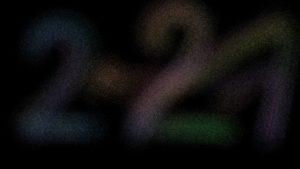###### Random Size Prisms Brush:
```void randomprisms() {
if (mousePressed) {
float d = dist(x, y, mouseX, mouseY);

if (d>stepSize) {
// gets the angle between the mouse and the location of the brush so that next point is drawn in the right intended location
angle = (float)Math.atan2(mouseY-y, mouseX-x);
for ( int i=0; i<8; i++) {
numX[i]+=random(0-stepSize/2, stepSize/2);
numY[i]+=random(0-stepSize/2, stepSize/2);
}

pushMatrix();
//
translate(x, y);
rotate(angle+random(-0.1, 0.1));
stroke(col);
strokeWeight(stepSize/4);
// if (frameCount%2 == 0) stroke(100);
// line(0, 0, 0, linelength*random(0.95, 1)*d/100);
rectMode(CENTER);
noFill();
rect(0, 0, linelength*random(0.95, 1)*d/100, linelength*random(0.95, 1)*d/100);

// noFill();
// beginShape();
// curveVertex(numX, numY);///////////////
// curveVertex(numX, numY);///////////////
// curveVertex(numX, numY);//mid
// curveVertex(numX, numY);///////////////
// curveVertex(numX, numY);// mid
// curveVertex(numX, numY);///////////////
// curveVertex(numX, numY);//mid
// curveVertex(numX, numY);///////////////
// curveVertex(numX, numY);//mid
// curveVertex(numX, numY);///////////////
// curveVertex(numX, numY);///////////////

// endShape();

popMatrix();

x=x+(float)Math.cos((angle))*stepSize;
y=y+(float)Math.sin((angle))*stepSize;
x=mouseX;
y=mouseY;
}
}
col = color(noise(100+0.01*frameCount)*255, noise(200+0.01*frameCount)*255, noise(300+0.01*frameCount)*255, 255);
}
```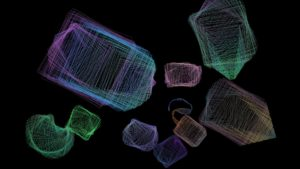```void dynamicShapes() {
if (mousePressed) {
float d = dist(x, y, mouseX, mouseY);

if (d>stepSize) {
//
lineModule = createShape();
stroke(col);
strokeWeight(10);
noFill();
lineModule.beginShape();

lineModule.vertex(100, 0); // first point

lineModule.vertex(0, 100);// /
lineModule.vertex(-100, 0);// \
lineModule.vertex(0, -100);// /
lineModule.vertex(100, 0);// \

lineModule.endShape();

// lineModule = createShape();
// stroke(col);
// strokeWeight(10);
// fill(col);
// lineModule.beginShape();
// lineModule.vertex(50, 75); // first point
// lineModule.bezierVertex(100, 100, 500, 100, 400, 300);
// lineModule.endShape();
//
// gets the angle between the mouse and the location of the brush so that next point is drawn in the right intended location
angle = (float)Math.atan2(mouseY-y, mouseX-x);

pushMatrix();
//
translate(mouseX, mouseY);
rotate((angle+PI));
shape(lineModule, 0, 0, d, noise(1+frameCount*0.0001)*10);
//
popMatrix();

x=x+(float)Math.cos((angle))*stepSize;
y=y+(float)Math.sin((angle))*stepSize;
}
}
col = color(noise(100+0.01*frameCount)*255, noise(200+0.01*frameCount)*255, noise(300+0.01*frameCount)*255, 255);
}

```
###### Text Brush:
```void textlines() {
if (mousePressed) {
float d = dist(x, y, mouseX, mouseY);
d= constrain(d, 60, 7000);

if (d>stepSize) {
// gets the angle between the mouse and the location of the brush so that next point is drawn in the right intended location
angle = (float)Math.atan2(mouseY-y, mouseX-x);
fontSize= fontSizeMin*60/d;
println("d: "+d);
font = createFont("Calibri", fontSize);

textFont(font, fontSize);
char newLetter = letters.charAt(counter);
stepSize = textWidth(newLetter);
fill(col);
//stroke(col);

pushMatrix();
//
translate(x, y);
rotate(angle);
text(newLetter, 0, 0);
//
popMatrix();

counter++;

if (counter>letters.length()-1) counter=0;

x = x + (float)Math.cos(angle)*stepSize;
y = y + (float)Math.sin(angle)*stepSize;
}
}
col = color(noise(100+0.01*frameCount)*255, noise(200+0.01*frameCount)*255, noise(300+0.01*frameCount)*255, 255);
}```
###### Rotating Brush:
```void rotatingLines() {

if (mousePressed) {
pushMatrix();
strokeWeight(3);
stroke(col);
//
translate(mouseX, mouseY);
line(0, 0, linelength, 0);
//
popMatrix();

angle +=1;
col = color(noise(100+0.01*frameCount)*255, noise(200+0.01*frameCount)*255, noise(300+0.01*frameCount)*255, 255);
linelength= int(noise(50+0.01*frameCount)*300);
}
}```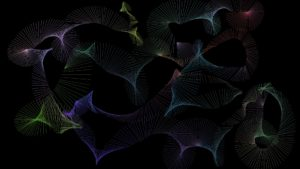#### Next Steps:

###### Processing:

1) Workout the user input method

2) Create the user interface and develop a collect pixels mechanism to restore the drawing after the color wheel appears when the user changes colors

3)Save the images and save files with people’s previous images to display images when the user saves the file.

###### Arduino:

1) Get readings from joyStick for color wheel and possibly brush.

2) Get readings from buttons for save and fader variable resistor for brush

## Final Project Idea: Generative Drawing

#### Description:

My idea is to make a drawing machine on Processing and to use Arduino-controlled Joy Sticks to control the mouse position on the screen.

The user would get to control the colors and save the image if desired.

If the user decides to save their generative art drawing, the 5 last saved drawings will show on the screen in a polaroid-like look.

#### Processing Inspiration:

I chose to make a generative art piece on processing because I feel that a drawing using a joystick can only get you so far without much practice, so I decided that a generative art algorithm would work better for this case.

These are some sources that I feel inspire my goal for the processing part of my project.

First design this is a randomly generated piece, but it is really close to what I thought of when I first thought of generative.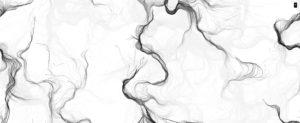Second design This is a drawing piece where the letters are drawn instead of ink at the position of the mouse and the size is decided by the speed of the movement of the mouse.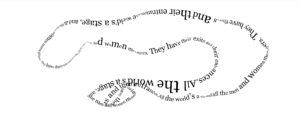Third design In this one the cursor is followed by randomly changing shape instead of a normal brush.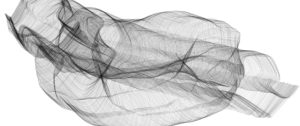Fourth design This, like the first, does not follow the mouse, it is just random instead-but follows a random path and is not completely random.

I think this might be nice to add behind any of the other designs with a lower opacity to give a smoky look behind the drawing.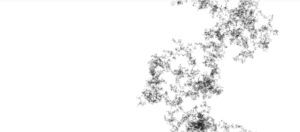#### Arduino:

My plan is to have 2 joysticks, one to control the mouse location and one to change the color of the drawing at a certain point.

I also plan to add push buttons to toggle values that will reset/save/show prev saved images.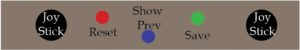This is the arrangement I have in mind at the moment

## Class Assignment: Arduino-Processing Communication

#### Exercise 1:

###### Processing:
```import processing.serial.*;
Serial myPort;
int xPos=0;
int yPos=0;
boolean onOff=false;
boolean onOff2=false;

void setup(){
size(960,720);
printArray(Serial.list());
String portname=Serial.list();
println(portname);
myPort = new Serial(this,portname,9600);
myPort.clear();
myPort.bufferUntil('\n');
}

void draw(){
background(255);
ellipse(xPos,yPos,30,30);
if (mousePressed){
if(mouseX<=width/2)
onOff2=true;
else
onOff=true;
}else{
onOff=onOff2=false;
}
}

void serialEvent(Serial myPort){
s=trim(s);
if (s!=null){
println(s);
int values[]=int(split(s,','));
if (values.length==2){
xPos=(int)map(values,0,1023,0, width);
yPos=height/2;
}
}
myPort.write(int(onOff)+","+int(onOff2)+"\n");
}```
###### Arduino:
```int left = 0;
int right = 0;

void setup() {
Serial.begin(9600);
Serial.println("0,0");
pinMode(3, OUTPUT);
pinMode(5, OUTPUT);
}

void loop() {
while (Serial.available()) {
right = Serial.parseInt();
left = Serial.parseInt();
digitalWrite(3, right);
digitalWrite(5, left);
delay(1);
delay(1);
Serial.print(sensor);
Serial.print(',');
Serial.println(sensor2);
}
}
}```

#### Exercise 2:

###### Processing:
```import processing.serial.*;
Serial myPort;
int xPos = 0;
int yPos = 0;
int onOff = 0;
int onOff2 = 0;
int brightness = 0;

void setup() {
size(960, 720);
printArray(Serial.list());
String portname = Serial.list();
println(portname);
myPort = new Serial(this, portname, 9600);
myPort.clear();
myPort.bufferUntil('\n');
}

void draw() {
background(255);
brightness = int(map(mouseY, 0, height, 0, 255));
ellipse(xPos, yPos, 30, 30);
if (mousePressed) {
if (mouseX <= width / 2) {
onOff2 = brightness;
} else {
onOff = brightness;
}
} else {
onOff = onOff2 = 0;
}
}

void serialEvent(Serial myPort) {
s = trim(s);
if (s != null) {
println(s);
int values[] = int(split(s, ','));
if (values.length == 2) {
xPos = (int)map(values, 0, 1023, 0, width);
//yPos=(int)map(values,0,1023,0, height);
yPos = mouseY;
}
}
myPort.write(int(onOff) + "," + int(onOff2) + "\n");
}
```
###### Arduino:
```int left = 0;
int right = 0;

void setup() {
Serial.begin(9600);
Serial.println("0,0");
pinMode(3, OUTPUT);
pinMode(5, OUTPUT);
}

void loop() {
while (Serial.available()) {
right = Serial.parseInt();
left = Serial.parseInt();
analogWrite(3, right);
analogWrite(5, left);
delay(1);
delay(1);
Serial.print(sensor);
Serial.print(',');
Serial.println(sensor2);
}
}
}
```

#### Exercise 3:

###### Processing:
```import processing.serial.*;
Serial myPort;

PVector velocity;
PVector gravity;
PVector position;
PVector acceleration;
PVector wind;
float drag = 0.99;
float mass = 50;
float hDampening;
int[] values;

int onoff, onoff2;

void setup() {
size(960, 720);
printArray(Serial.list());

noFill();
position = new PVector(width/2, 0);
velocity = new PVector(0, 0);
acceleration = new PVector(0, 0);
gravity = new PVector(0, 0.5*mass);
wind = new PVector(0, 0);
hDampening=map(mass, 15, 80, .98, .96);

String portname=Serial.list();
println(portname);
myPort = new Serial(this, portname, 9600);
myPort.clear();
myPort.bufferUntil('\n');
}

void draw() {
background(255);
applyForce(wind);
applyForce(gravity);
velocity.mult(drag);
acceleration.mult(0);
ellipse(position.x, position.y, mass, mass);
if (position.y > height-mass/2) {
velocity.y *= -0.9;  // A little dampening when hitting the bottom
position.y = height-mass/2;
}

if (velocity.y!=0) {
onoff=1;
onoff2=0;
} else {
onoff=0;
onoff2=1;
}
}

void applyForce(PVector force) {
// Newton's 2nd law: F = M * A
// or A = F / M
PVector f = PVector.div(force, mass);
}

void serialEvent(Serial myPort) {
s=trim(s);
if (s!=null) {
println(s);
values=int(split(s, ','));
if (values.length==3) {
if (values==1) {
wind.x=-2;
}
if (values==1) {
wind.x=2;
}
if (values==1) {
mass=random(15, 80);
position.y=-mass;
velocity.mult(0);
}
if (values==0 && values==0) {
wind.x=0;
velocity.x*=hDampening;
}
}
}
myPort.write(onoff+ ","+onoff2+"\n");
}

```
###### Arduino:
```int left = 0;
int right = 0;

void setup() {
Serial.begin(9600);
Serial.println("0,0,0");
pinMode(3, OUTPUT);
pinMode(5, OUTPUT);
pinMode(A0, INPUT);
pinMode(A1, INPUT);
pinMode(A2, INPUT);
}

void loop() {
while (Serial.available()) {
right = Serial.parseInt();
left = Serial.parseInt();
digitalWrite(3, right);
digitalWrite(5, left);
delay(1);
delay(1);
delay(1);
Serial.print(button);
Serial.print(',');
Serial.print(button2);
Serial.print(',');
Serial.println(button3);
}
}
}
```

## Week 9 Refill

#### Description:

I decided, after a struggle of thought, to make a simple water refill reminder. Drinking water is crucial and many people tend to try to forget. So I made this simple water that detects the weight of the bottle and knows how much water is left in the bottle/cup using a force sensor.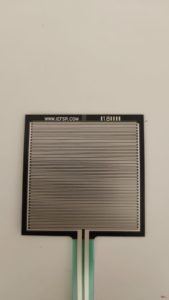using the force sensor on its own did not give a range of weight enough for the water weight detector. so I decided to place the force sensor on a sponge.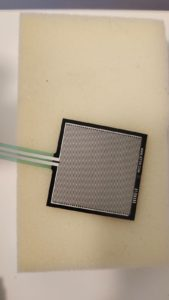I also used an on/off button that turns the circuit on when pressed and off when pressed once more

I also used 4 red lights that are more likely to capture someone’s attention and remind them to refill their water bottle when it’s empty.

and I used 4 blue led lights, more of the blue lights light up the more water there is in the bottle.

this is how my circuit looks: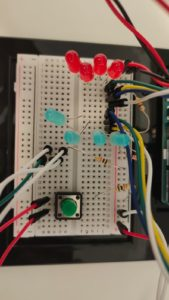the last set of wires that are neither connected to the button or the less is connected to the force sensor.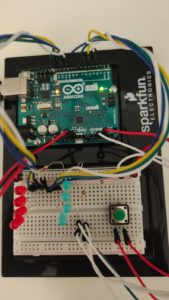#### video:

the video shows how the circuit works:

#### Code:

```const int outs[] = {3, 5, 6, 9,
10, 11, 12, 13
};
const int ins[] = {A2, A3};
int var, var2, b, r, maxx = 0;

long timer;
bool turnon = 0, curr, prev;

void setup() {
Serial.begin(9600);
for (int i = 0; i < sizeof(outs); i++) {
pinMode(outs[i], OUTPUT);
}
Serial.begin(9600);
for (int i = 0; i < sizeof(ins); i++) {
pinMode(ins[i], INPUT);
}
}

void loop() {
if (curr != prev && curr == 1) {
turnon = !turnon;
}
timer = millis();
var = map(var, 0, 500, 0, 255);
var2 = constrain(var, 250, 255);
Serial.print(" on: ");
Serial.print(turnon);
Serial.print(" curr: ");
Serial.print(curr);
Serial.print(" prev: ");
Serial.println(prev);

if (turnon == 1) {
on();
}
else {
for (int i = 0; i < 8; i++) {
analogWrite(outs[i], 0);
}
}
//

}

void redled(int n) {
if (timer % (n * 4) < (n * 1)) {
analogWrite(outs, var2);
analogWrite(outs, 0);
analogWrite(outs, 0);
analogWrite(outs, 0);
}
else if (timer % (n * 4) < (n * 2)) {
analogWrite(outs, 0);
analogWrite(outs, var2);
analogWrite(outs, 0);
analogWrite(outs, 0);
}
else if (timer % (n * 4) < (n * 3)) {
analogWrite(outs, 0);
analogWrite(outs, 0);
analogWrite(outs, var2);
analogWrite(outs, 0);
}
else {
analogWrite(outs, 0);
analogWrite(outs, 0);
analogWrite(outs, 0);
analogWrite(outs, var2);
}
}

void on() {

if (var < 20) {
b = -1;
r = 4;
}
else if (var < 140) {
b = 0;
r = 0;
}
else if (var < 260) {
b = 1;
r = 0;
}
else if (var < 380) {
b = 2;
r = 0;
}
else {
b = 3;
r = 0;
}

// there is water
if (r == 0) {
for (int i = 0; i < b + 1; i++) {
analogWrite(outs[i], var2);
}
for (int i = 7; i > b; i--) {
analogWrite(outs[i], 0);
}
}
else {
for (int i = 0; i < 4; i++) {
analogWrite(outs[i], 0);
}
redled(1000);

}
}```

## Week 8 The Switch

#### Description:

I have always been the worst when it came to forgetting different things at different places. Forgetting my card at the most random places was getting me locked out of my room more than I would have liked. So I decided to make a switch that reminds you to put your card back in its place sometime out.

I started by placing a switch in the circuit and making it work using a normal push-button switch. once I finished the circuit I replaced one end of the switch with a wire connected to the card and the other end with a wire connected to a cardholder that is to be stuck to a phone.

I connected the wires to copper tape that I stuck on contacting sides of the card and the cardholder so that when the card is in place, the switch is closed.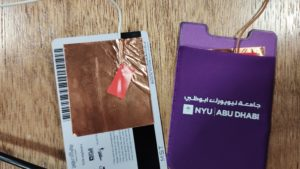I used a yellow led light for the normal time when the card is placed in its location. when you remove the card from the cardholder, a timer starts, when the time span in the code ends, the red light starts to blink to remind you to put the card back in.

#### IMAGES:

Whole Circuit: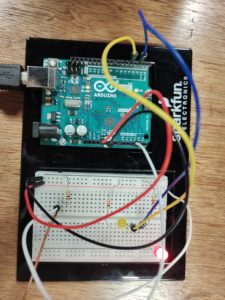#### CODE:

```long mytime=500, timer = 0;
const int outs[] = {2, 4};
const int ins = A2;
int curr = 0, prev=0;
bool flip=false;

void setup() {
// put your setup code here, to run once:
Serial.begin(9600);
for (int i = 0; i < sizeof(outs); i++) {
pinMode(outs[i], OUTPUT);
}
pinMode(ins, INPUT);
}

void loop() {
curr = digitalRead(ins);// <500, <900, >900
if (curr == 0) {
Serial.print(timer);
Serial.print(" ");
Serial.println(mytime);
if (mytime == timer) {
flip=!flip;
}
digitalWrite(outs, flip);
digitalWrite(outs, LOW);
}
else{
timer+=1;
digitalWrite(outs, LOW);
digitalWrite(outs, LOW);
}
}
else {
{
timer=0;
digitalWrite(outs, HIGH);
digitalWrite(outs, LOW);
}
}
}```

#### Overview:

Finally, it’s here.

The purpose of this game is for the student, skeleton in the picture, to collect all the questions and then collect the final paper to get the full grade for their exam.

#### IDEA(details):

##### Background:

Originally, I intended to have clouds as the background with the stars, but even when I used images instead of pixels, the game’s frameRate dropped and the game was barely functioning so I settled for a clear starry night look.

I choose this because:

firstly, I have really sensitive eyes, and I find it easier on my eye to look at a dark screen.

Secondly, I am a night person, night always looks better ;).

For the stars, I used overlapping circles to give a faded look, which resembles actual stars more than normal circles would.

###### Code:
```class Bg {
float[] x = new float;
float[] y = new float;
Bg() {
for (int i=0; i<50; i++) {
x[i] = random(0, widthh);
y[i] = random(0, heightt);
}
}

void drawBG() {
if (frameCount%50==0) {
for (int i=0; i<50; i++) {
x[i] = random(0, width);
y[i] = random(0, height);
}
}
background(50, 50, 80);
for (int i=0; i<50; i++) {
for (int j=0; j<10; j++) {
noStroke();
fill(255, 25);
ellipse(x[i], y[i], 1+j, 1+j);
}
}
}
}```

##### Creature:

Instead of making repetitive classes, I used class inheritance, where I made the Superclass, Creatures, for all the live items in the game.

###### Code:
```public class Creature {

// Attributes
PVector pos;
PVector speed;
boolean alive;
float ground;
PVector size;
PVector imgSize;
int numFrames;
PImage imgs;
PImage[] img;
int frame;
boolean dir; //left = 0 right = 1
boolean secondJump = false;

public Creature(float x, float  y, float  r, float  g, PImage _img, float  w, float  h, int  num_frames) {
pos= new PVector(x, y);
speed= new PVector(0, 0);
alive = true;
ground = height-g;
numFrames = num_frames;
imgSize = new PVector(_img.width/num_frames, _img.height);
size = new PVector (r, imgSize.y*(r/imgSize.x));
imgs = _img;
img = new PImage[numFrames];
for (int i=0; i<numFrames; i++) {
img[i] = imgs.get(
int((imgSize.x)*i),
0,
int(imgSize.x),
int(imgSize.y));
}
// for (int y=0; y < 4; y++) {
//   for (int x=0; x< 12; x++) {
//     sprites[y][x] = spritesheet.get(x*w, y*h, w, h);
//   }
// }
frame = 0;
dir = true;
}

void gravity() {
if (pos.y<ground-size.y+10  ||pos.y<ground-size.y ) {
speed.y+=0.2;
} else {
speed.y=0;
pos.y = constrain(pos.y, ground-size.y, ground-size.y+10);
secondJump=false;
}
for (int p=game.plats.length-1; p>=0; p--) {
if ((pos.y+size.y <=game.plats[p].pos.y+15)//height
&& pos.x+size.x/3>=game.plats[p].pos.x//right
&& pos.x-size.x/3  <= game.plats[p].pos.x+game.plats[p].pWidth) {//left
ground = game.plats[p].pos.y;
break;
} else {
ground = heightt-game.ground;
}
}
}

void update() {
gravity();
pos.x += speed.x;
pos.y += speed.y;
}
void display() {
update();
// println(this.getClass().getName());
if (dir && alive) {
scale(1, 1);
image(img[frame], pos.x, pos.y, size.x, size.y);
} else if (!dir && alive) {
if (this.getClass().getName()=="Midterm\$Player") {
pushMatrix();
scale(-1, 1);
image(img[frame], -pos.x, pos.y, size.x, size.y);
popMatrix();
} else if (this.getClass().getName()=="Midterm\$Monster") {
image(img[frame], pos.x, pos.y, size.x, size.y);
}
}
}
}```
##### Player:

For the player, I choose the skeleton, because obviously, we are all dead during midterm and final seasons. That also gave me the idea of the name “dead student“.

Sprite: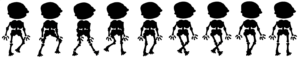With the player, I struggled a little with the jumps. I wanted to only allow double jumps, which I thought was the case until my roommate tried it and I realized it didn’t. I tried to fix it, but that only made it worse, so I left it as it was when my roommate tried it. (I mean what works, works right?)

###### Code:
```class Player extends Creature {
boolean left;
boolean up;
boolean right;
SoundFile jump;
int count;
Player(float x, float  y, float  r, float  g, PImage _img, float  w, float  h, int  num_frames, PApplet sketch) {
super(x, y, r, g, _img, w, h, num_frames);
left = false;
up = false;
right = false;
jump = new SoundFile(sketch, "jump.wav");
count = frameCount;
}

void update() {
gravity();
//====================
if (left==true) {
speed.x=-3;
dir=false;
} else if (right==true) {
speed.x=3;
dir=true;
} else {
speed.x=0;
}
//====================
if (up==true && pos.y>=ground-size.y &&secondJump==false) {
jump.play();
speed.y = -6;
} else if (up==true && speed.y<0 && secondJump==true && pos.y<ground-size.y) {
jump.play();
speed.y = -8;
secondJump=false;
}
pos.x +=speed.x;
pos.y +=speed.y;
if (frameCount%5 == 0 && speed.x !=0 && speed.y ==0) {
frame= (frame+1)%numFrames;
} else if (speed.x==0) {
frame=8;
}

//====================
if (pos.x<=0) {
pos.x = 0;
}
//====================
if (pos.x+size.x>= widthh) {
pos.x = widthh- size.x;
} else if (pos.x<size.x) {
pos.x = size.x;
}
//====================
for (int i =0; i<game.myMonsters.length; i++) {
float numME = pow((pow(size.x, 2)+(pow(size.y, 2))), 0.5);
float numthem = pow((pow(game.myMonsters[i].size.x, 2)+(pow(game.myMonsters[i].size.y, 2))), 0.5);

if (game.myMonsters[i].distance(this)<=numME/2.5+numthem/2.5 && game.myMonsters[i].alive) {
if (speed.y>0) {
game.myMonsters[i].alive=false;
game.myMonsters[i].sound2.play();
} else {
game.myMonsters[i].sound1.play();
alive = false;
}
}
}
for (int i =0; i<game.myQuestions.length; i++) {
if (game.myQuestions[i].distance(this)<=size.x/2+game.myQuestions[i].size.x/2 && game.myQuestions[i].alive) {
game.score++;
game.myQuestions[i].sound.play();
game.myQuestions[i].alive = false;
}
}
if (game.myfinal.distance(this)<=size.x/2+game.myfinal.size.x/2 && game.myfinal.alive) {
game.score+=5;
game.myfinal.sound.play();
game.myfinal.alive = false;
game.won= true;
}
}
}```
##### Monsters:

Finding Sprites for the monsters was not easy. I was trying to find monsters that represent the distractions we have while studying. So instead of finding different monsters, I made my own using emojis and clip art images.

I used the facial features from the emojis and the body of what I think represents distractions. I made them alternate between an Innocent look and an evil look because distractions usually don’t look so bad.

Here are the sprites:

Monster 1, Phone:Monster 2, Thoughts:Monster 3, Anxiety: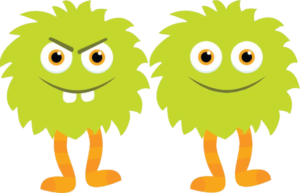###### Code:
```class Monster extends Creature {
SoundFile sound1, sound2;
float xleft, xright;
Monster(float x, float  y, float  r, float  g, PImage _img, float  w, float  h, int  num_frames, float x_left, float x_right, PApplet sketch) {
super(x, y, r, g, _img, w, h, num_frames);

speed.x=random(0.3,0.9);
speed.y=0;
xleft = x_left;
xright = x_right;
sound1 = new SoundFile(sketch, "eat.wav");
sound2 = new SoundFile(sketch, "kill.wav");
}

void update() {
gravity();
if (pos.x<=xleft) {
dir = true;
frame= (frame+1)%numFrames;
speed.x*=-1;
} else if (pos.x>=xright) {
dir = false;
frame= (frame+1)%numFrames;
speed.x*=-1;
}
pos.x +=speed.x;
pos.y +=speed.y;
}

float distance(Player target) {
return dist(this.pos.x+size.x/2, this.pos.y+size.y/2, target.pos.x+target.size.x/2, target.pos.y+target.size.y/2);
}
}```
##### Questions and Final:

For this one, I thought it was better to have a bouncy still image, as it was the only one that was completely dead. I used the same class for both as they had the same charactaristics.

Sprites:

Questions:Final: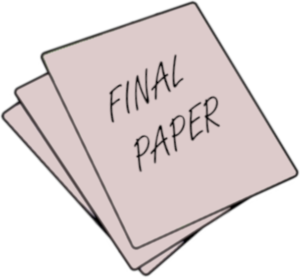###### Code:
```class Question extends Creature {
SoundFile sound;
Question(float x, float  y, float  r, float  g, PImage _img, float  w, float  h, int  num_frames, PApplet sketch) {
super(x, y, r, g, _img, w, h, num_frames);
speed.x=0;
speed.y=-2;
sound = new SoundFile(sketch, "token.mp3");
}

void update() {
gravity();
if (speed.y==0) {
speed.y=-2;
}

pos.x +=speed.x;
pos.y +=speed.y;
}

float distance(Player target) {
return dist(this.pos.x, this.pos.y, target.pos.x, target.pos.y);
}
}```

##### Game:

For the full game, I made a game class that allows me to create a game object whenever I want to start the game. This helps with restarting and waiting for the user to press a key to start; as I just create a game object if the player is dead and the user presses enter at the welcom screen.

My game class only had the creation of all the game objects and the display call for them.

###### Code:
```class Game {

//
int ground = 100;
public int score = 0;
boolean won;

Player myplayer;
Platforms[] plats = new Platforms;
Platforms groundd;
int platLocH[] = new int;
int platLocW[] = new int;

Monster[] myMonsters = new Monster;

Question[] myQuestions = new Question;

Question myfinal;

Game(PApplet sketch, PImage playerImg, PImage[] monsters, PImage questionIMG, PImage finalIMG) {
// creating ground
groundd = new Platforms(0, heightt-ground, widthh, ground, 0);

// creating platforms
for (int i=0; i<plats.length; i++) {
platLocH[i] = (heightt-255)-i*155;
platLocW[i] = int(random(0, widthh-500));
plats[i]= new Platforms(platLocW[i], platLocH[i], 600, heightt/25, 15);
}

// creating Monsters(1@ground & 4 at plats 0-3)
int n;
float m;
for (int i=4; i>-1; i--) {
n = int(random(0, 3));
myMonsters[i]= new Monster(platLocW[i]+60, platLocH[i]-60*(monsters[n].width/monsters[n].height)-10, 60, ground, monsters[n], monsters[n].width, monsters[n].height, 2, platLocW[i]+random(40, 50), platLocW[i]+random(160, 220), sketch);
}
n = int(random(0, 3));
m = random(widthh/2, widthh-450);
myMonsters = new  Monster(m+60, heightt-ground, 60, ground, monsters[n], monsters[n].width, monsters[n].height, 2, m+random(40, 50), m+random(160, 220), sketch);

// creating Questions
for (int i=3; i>-1; i--) {
myQuestions[i]=new Question(platLocW[i]+400, platLocH[i]-60*(questionIMG.width/questionIMG.height)-50, 60, ground, questionIMG, questionIMG.width, questionIMG.height, 1, sketch);
}
myQuestions=new Question(m+400, heightt-ground, 60, ground, questionIMG, questionIMG.width, questionIMG.height, 1, sketch);

// creating final
myfinal = new Question(platLocW+400, platLocH-80*(finalIMG.width/finalIMG.height)-50, 80, ground, finalIMG, finalIMG.width, finalIMG.height, 1, sketch);
myfinal.alive=false;

// creating Player
myplayer = new Player(20, height-ground-180, 70, ground, playerImg, playerImg.width, playerImg.height, 9, sketch);
won = false;
}

void main() {
for (int i = 0; i<5; i++) {
plats[i].display();
if (myQuestions[i].alive) {
myQuestions[i].display();
}
}
groundd.display();
myplayer.display();
for (int i=0; i<6; i++) {
if (myMonsters[i].alive) {
myMonsters[i].display();
}
}
if (score == myQuestions.length) {
myfinal.alive = true;
myfinal.display();
}
}
}```

##### Start and end screens and Key presses:

I made functions to display text at the start and end of each game. This allowed me to easily call either whenever needed.

I reset my game variables in the end screen functions, but that is just to avoid unexpected errors.

```void startSc() {
textFont(f, 80);
fill(255);
textAlign(CENTER);
textFont(f, 40);
text("press enter to start", widthh/2, heightt/2-10);

textFont(f, 20);
text("Collect the questions to get to final paper", widthh/2, heightt/2+100);
text("You can kill monsters by jumping on them", widthh/2, heightt/2+130);
text("Please use left, right, and up arrows to play", widthh/2, heightt/2+190);
}

void endSc() {
if (game.won) {
textFont(f, 40);
fill(255);
textAlign(CENTER);
text("YOU WON!!", widthh/2, heightt/2);
text(String.valueOf(game.score)+" /10", widthh/2, heightt/2+100);
text("CONGRATS!!", widthh/2, heightt/2+150);
textFont(f, 20);
text("press r to reset", widthh/2, heightt/2+200);
} else {
for (int i=0; i<game.myMonsters.length; i++) {
game.myMonsters[i].alive=true;
}
for (int i=0; i<game.myQuestions.length; i++) {
game.myQuestions[i].alive=true;
}
textFont(f, 40);
fill(255);
textAlign(CENTER);
text("YOU DIED", widthh/2, heightt/2);
text(String.valueOf(game.score)+" /10", widthh/2, heightt/2+100);
textFont(f, 20);
text("press r to reset", widthh/2, heightt/2+150);
}
}```
###### Key Pressed/Released Code:

I created the game when the user presses enter;

```void keyPressed() {
if (keyCode == 10 && started==false) {//enter
started = true;
game = new Game(this, playerimg, monsters, question, finall);
} else if (keyCode==82 && started==true) {//r-> reset
started=false;
startSc();
} else if (started==true && game.won==false) {
if (keyCode == LEFT) {
game.myplayer.left = true;
}
if (keyCode==RIGHT) {
game.myplayer.right = true;
}
if (keyCode==UP) {
game.myplayer.up = true;
}
}
}

void keyReleased() {
if (started==true) {
if (keyCode == LEFT) {
game.myplayer.left = false;
}
if (keyCode==RIGHT) {
game.myplayer.right = false;
}
if (keyCode==UP) {
game.myplayer.up = false;
game.myplayer.secondJump=true;
}
}
}
```

#### Full Code:

```import processing.sound.*;

int heightt= 1000;
int widthh = 1400;
PFont f;

class Platforms {

PVector pos; //mid point
float pHeight;
float pWidth;
float widths;

Platforms(float x, float y, float w, float h, float r) {
pos = new PVector(x, y);
pHeight = h;
pWidth = w;
}

void display() {
noStroke();
rectMode(CORNER);
fill(30);
}
}

class Bg {
float[] x = new float;
float[] y = new float;
Bg() {
for (int i=0; i<50; i++) {
x[i] = random(0, widthh);
y[i] = random(0, heightt);
}
}

void drawBG() {
if (frameCount%50==0) {
for (int i=0; i<50; i++) {
x[i] = random(0, width);
y[i] = random(0, height);
}
}
background(50, 50, 80);
for (int i=0; i<50; i++) {
for (int j=0; j<10; j++) {
noStroke();
fill(255, 25);
ellipse(x[i], y[i], 1+j, 1+j);
}
}
}
}

public class Creature {

// Attributes
PVector pos;
PVector speed;
boolean alive;
float ground;
PVector size;
PVector imgSize;
int numFrames;
PImage imgs;
PImage[] img;
int frame;
boolean dir; //left = 0 right = 1
boolean secondJump = false;

public Creature(float x, float  y, float  r, float  g, PImage _img, float  w, float  h, int  num_frames) {
pos= new PVector(x, y);
speed= new PVector(0, 0);
alive = true;
ground = height-g;
numFrames = num_frames;
imgSize = new PVector(_img.width/num_frames, _img.height);
size = new PVector (r, imgSize.y*(r/imgSize.x));
imgs = _img;
img = new PImage[numFrames];
for (int i=0; i<numFrames; i++) {
img[i] = imgs.get(
int((imgSize.x)*i),
0,
int(imgSize.x),
int(imgSize.y));
}
// for (int y=0; y < 4; y++) {
//   for (int x=0; x< 12; x++) {
//     sprites[y][x] = spritesheet.get(x*w, y*h, w, h);
//   }
// }
frame = 0;
dir = true;
}

void gravity() {
if (pos.y<ground-size.y+10  ||pos.y<ground-size.y ) {
speed.y+=0.2;
} else {
speed.y=0;
pos.y = constrain(pos.y, ground-size.y, ground-size.y+10);
secondJump=false;
}
for (int p=game.plats.length-1; p>=0; p--) {
if ((pos.y+size.y <=game.plats[p].pos.y+15)//height
&& pos.x+size.x/3>=game.plats[p].pos.x//right
&& pos.x-size.x/3  <= game.plats[p].pos.x+game.plats[p].pWidth) {//left
ground = game.plats[p].pos.y;
break;
} else {
ground = heightt-game.ground;
}
}
}

void update() {
gravity();
pos.x += speed.x;
pos.y += speed.y;
}
void display() {
update();
// println(this.getClass().getName());
if (dir && alive) {
scale(1, 1);
image(img[frame], pos.x, pos.y, size.x, size.y);
} else if (!dir && alive) {
if (this.getClass().getName()=="Midterm\$Player") {
pushMatrix();
scale(-1, 1);
image(img[frame], -pos.x, pos.y, size.x, size.y);
popMatrix();
} else if (this.getClass().getName()=="Midterm\$Monster") {
image(img[frame], pos.x, pos.y, size.x, size.y);
}
}
}
}

class Player extends Creature {
boolean left;
boolean up;
boolean right;
SoundFile jump;
int count;
Player(float x, float  y, float  r, float  g, PImage _img, float  w, float  h, int  num_frames, PApplet sketch) {
super(x, y, r, g, _img, w, h, num_frames);
left = false;
up = false;
right = false;
jump = new SoundFile(sketch, "jump.wav");
count = frameCount;
}

void update() {
gravity();
//====================
if (left==true) {
speed.x=-3;
dir=false;
} else if (right==true) {
speed.x=3;
dir=true;
} else {
speed.x=0;
}
//====================
if (up==true && pos.y>=ground-size.y &&secondJump==false) {
jump.play();
speed.y = -6;
} else if (up==true && speed.y<0 && secondJump==true && pos.y<ground-size.y) {
jump.play();
speed.y = -8;
secondJump=false;
}
pos.x +=speed.x;
pos.y +=speed.y;
if (frameCount%5 == 0 && speed.x !=0 && speed.y ==0) {
frame= (frame+1)%numFrames;
} else if (speed.x==0) {
frame=8;
}

//====================
if (pos.x<=0) {
pos.x = 0;
}
//====================
if (pos.x+size.x>= widthh) {
pos.x = widthh- size.x;
} else if (pos.x<size.x) {
pos.x = size.x;
}
//====================
for (int i =0; i<game.myMonsters.length; i++) {
float numME = pow((pow(size.x, 2)+(pow(size.y, 2))), 0.5);
float numthem = pow((pow(game.myMonsters[i].size.x, 2)+(pow(game.myMonsters[i].size.y, 2))), 0.5);

if (game.myMonsters[i].distance(this)<=numME/2.5+numthem/2.5 && game.myMonsters[i].alive) {
if (speed.y>0) {
game.myMonsters[i].alive=false;
game.myMonsters[i].sound2.play();
} else {
game.myMonsters[i].sound1.play();
alive = false;
}
}
}
for (int i =0; i<game.myQuestions.length; i++) {
if (game.myQuestions[i].distance(this)<=size.x/2+game.myQuestions[i].size.x/2 && game.myQuestions[i].alive) {
game.score++;
game.myQuestions[i].sound.play();
game.myQuestions[i].alive = false;
}
}
if (game.myfinal.distance(this)<=size.x/2+game.myfinal.size.x/2 && game.myfinal.alive) {
game.score+=5;
game.myfinal.sound.play();
game.myfinal.alive = false;
game.won= true;
}
}
}

class Monster extends Creature {
SoundFile sound1, sound2;
float xleft, xright;
Monster(float x, float  y, float  r, float  g, PImage _img, float  w, float  h, int  num_frames, float x_left, float x_right, PApplet sketch) {
super(x, y, r, g, _img, w, h, num_frames);

speed.x=random(0.3,0.9);
speed.y=0;
xleft = x_left;
xright = x_right;
sound1 = new SoundFile(sketch, "eat.wav");
sound2 = new SoundFile(sketch, "kill.wav");
}

void update() {
gravity();
if (pos.x<=xleft) {
dir = true;
frame= (frame+1)%numFrames;
speed.x*=-1;
} else if (pos.x>=xright) {
dir = false;
frame= (frame+1)%numFrames;
speed.x*=-1;
}
pos.x +=speed.x;
pos.y +=speed.y;
}

float distance(Player target) {
return dist(this.pos.x+size.x/2, this.pos.y+size.y/2, target.pos.x+target.size.x/2, target.pos.y+target.size.y/2);
}
}

class Question extends Creature {
SoundFile sound;
Question(float x, float  y, float  r, float  g, PImage _img, float  w, float  h, int  num_frames, PApplet sketch) {
super(x, y, r, g, _img, w, h, num_frames);
speed.x=0;
speed.y=-2;
sound = new SoundFile(sketch, "token.mp3");
}

void update() {
gravity();
if (speed.y==0) {
speed.y=-2;
}

pos.x +=speed.x;
pos.y +=speed.y;
}

float distance(Player target) {
return dist(this.pos.x, this.pos.y, target.pos.x, target.pos.y);
}
}

class Game {

//
int ground = 100;
public int score = 0;
boolean won;

Player myplayer;
Platforms[] plats = new Platforms;
Platforms groundd;
int platLocH[] = new int;
int platLocW[] = new int;

Monster[] myMonsters = new Monster;

Question[] myQuestions = new Question;

Question myfinal;

Game(PApplet sketch, PImage playerImg, PImage[] monsters, PImage questionIMG, PImage finalIMG) {
// creating ground
groundd = new Platforms(0, heightt-ground, widthh, ground, 0);

// creating platforms
for (int i=0; i<plats.length; i++) {
platLocH[i] = (heightt-255)-i*155;
platLocW[i] = int(random(0, widthh-500));
plats[i]= new Platforms(platLocW[i], platLocH[i], 600, heightt/25, 15);
}

// creating Monsters(1@ground & 4 at plats 0-3)
int n;
float m;
for (int i=4; i>-1; i--) {
n = int(random(0, 3));
myMonsters[i]= new Monster(platLocW[i]+60, platLocH[i]-60*(monsters[n].width/monsters[n].height)-10, 60, ground, monsters[n], monsters[n].width, monsters[n].height, 2, platLocW[i]+random(40, 50), platLocW[i]+random(160, 220), sketch);
}
n = int(random(0, 3));
m = random(widthh/2, widthh-450);
myMonsters = new  Monster(m+60, heightt-ground, 60, ground, monsters[n], monsters[n].width, monsters[n].height, 2, m+random(40, 50), m+random(160, 220), sketch);

// creating Questions
for (int i=3; i>-1; i--) {
myQuestions[i]=new Question(platLocW[i]+400, platLocH[i]-60*(questionIMG.width/questionIMG.height)-50, 60, ground, questionIMG, questionIMG.width, questionIMG.height, 1, sketch);
}
myQuestions=new Question(m+400, heightt-ground, 60, ground, questionIMG, questionIMG.width, questionIMG.height, 1, sketch);

// creating final
myfinal = new Question(platLocW+400, platLocH-80*(finalIMG.width/finalIMG.height)-50, 80, ground, finalIMG, finalIMG.width, finalIMG.height, 1, sketch);
myfinal.alive=false;

// creating Player
myplayer = new Player(20, height-ground-180, 70, ground, playerImg, playerImg.width, playerImg.height, 9, sketch);
won = false;
}

void main() {
for (int i = 0; i<5; i++) {
plats[i].display();
if (myQuestions[i].alive) {
myQuestions[i].display();
}
}
groundd.display();
myplayer.display();
for (int i=0; i<6; i++) {
if (myMonsters[i].alive) {
myMonsters[i].display();
}
}
if (score == myQuestions.length) {
myfinal.alive = true;
myfinal.display();
}
}
}

void startSc() {
textFont(f, 80);
fill(255);
textAlign(CENTER);
textFont(f, 40);
text("press enter to start", widthh/2, heightt/2-10);

textFont(f, 20);
text("Collect the questions to get to final paper", widthh/2, heightt/2+100);
text("You can kill monsters by jumping on them", widthh/2, heightt/2+130);
text("Please use left, right, and up arrows to play", widthh/2, heightt/2+190);
}

void endSc() {
if (game.won) {
textFont(f, 40);
fill(255);
textAlign(CENTER);
text("YOU WON!!", widthh/2, heightt/2);
text(String.valueOf(game.score)+" /10", widthh/2, heightt/2+100);
text("CONGRATS!!", widthh/2, heightt/2+150);
textFont(f, 20);
text("press r to reset", widthh/2, heightt/2+200);
} else {
for (int i=0; i<game.myMonsters.length; i++) {
game.myMonsters[i].alive=true;
}
for (int i=0; i<game.myQuestions.length; i++) {
game.myQuestions[i].alive=true;
}
textFont(f, 40);
fill(255);
textAlign(CENTER);
text("YOU DIED", widthh/2, heightt/2);
text(String.valueOf(game.score)+" /10", widthh/2, heightt/2+100);
textFont(f, 20);
text("press r to reset", widthh/2, heightt/2+150);
}
}

Game game;
PImage playerimg;
PImage[] monsters = new PImage;
PImage question, finall, bgImg;
Bg bckgrnd;
boolean started;
SoundFile backgroundSound;

backgroundSound = new SoundFile(this, "bg.wav");
}

void setup() {
size(1400, 1000);
f = createFont("Courier New", 32);
started = false;
bckgrnd = new Bg();
}

void draw() {
background(100);
bckgrnd.drawBG();
if (!backgroundSound.isPlaying()) {
backgroundSound.play();
}

if (started) {
if (game.myplayer.alive && game.won==false) {
game.main();
textFont(f, 28);
fill(255);
textAlign(LEFT);
} else {
endSc();
}
} else {
startSc();
}
}

void keyPressed() {
if (keyCode == 10 && started==false) {//enter
started = true;
game = new Game(this, playerimg, monsters, question, finall);
} else if (keyCode==82 && started==true) {//r-> reset
started=false;
startSc();
} else if (started==true && game.won==false) {
if (keyCode == LEFT) {
game.myplayer.left = true;
}
if (keyCode==RIGHT) {
game.myplayer.right = true;
}
if (keyCode==UP) {
game.myplayer.up = true;
}
}
}

void keyReleased() {
if (started==true) {
if (keyCode == LEFT) {
game.myplayer.left = false;
}
if (keyCode==RIGHT) {
game.myplayer.right = false;
}
if (keyCode==UP) {
game.myplayer.up = false;
game.myplayer.secondJump=true;
}
}
}
```

#### Inspiration:

There is a game played a while back that I really liked, and as recreating that game will take much longer than the time I have now, I got an idea that is similar in a way, but simpler.

The game I got inspired by is called HUE.In this game, hue needs to save his mom by collecting pieces of the color ring in the picture above.

#### My Idea:

My idea, similarly, the student needs to collect a few questions and the exam to pass.

Game Parts:

– The player/student needs to collect all the questions on the screen and get to the final before the timer ends.

– If the student collects all the questions, they can collect the final exam paper and get the full grade on the exam. Otherwise, they get points for the questions they collected.

-each question is guarded by a monster(monsters are inspired by the monsters that face students like anxiety, procrastination, and distractions).

This is a rough draft of what the game is supposed to look like: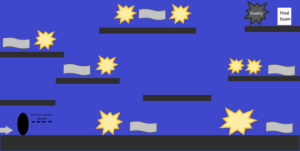#### Progress:

I started with the background. Instead of using a still image, I used loadPixels() to make the background look something like a night sky.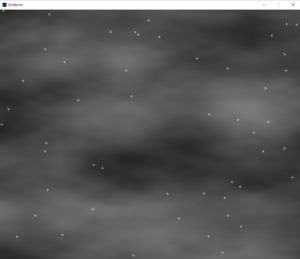##### Code:

```class Bg {
float[] x = new float;
float[] y = new float;
Bg() {
for (int i=0; i<50; i++) {
x[i] = random(0, widthh);
y[i] = random(0, heightt);
}
}
void drawBG() {
for (int y=0; y<heightt; y++) {
for (int x=0; x<widthh; x++) {
float n = noise(x*.002, y*.005);
//n = frameCount%width+n;
//n= map(n,0,width,0,255);
pixels[x+y*width]=color(n*150);
}
}
updatePixels();

if (frameCount%5==0) {
for (int i=0; i<50; i++) {
x[i] = random(0, width);
y[i] = random(0, height);
}
}
for (int i=0; i<50; i++) {
for (int j=0; j<10; j++) {
noStroke();
fill(255, 25);
ellipse(x[i], y[i], 1+j, 1+j);
}
}
}
}
```

I added platforms, which the questions and monsters will be on at random, saved, x-locations: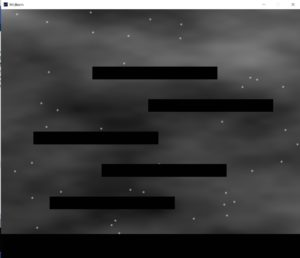##### Code:
```class Platforms {

PVector pos; //mid point
float pHeight;
float pWidth;
float widths;

Platforms(float x, float y, float w, float h) {
pos = new PVector(x, y);
//println(pos.x, pos.y);
pHeight = h;
pWidth = w;
}

void display() {
stroke(0);
//noStroke();
rectMode(CORNER);
fill(1);
rect(pos.x, pos.y, pWidth, pHeight);
}
// float distance(Objects other){

// }
}```

My code is mainly classes for each thing on the screen and one for the whole game that controls everything.

I made one creature’s class that will be a superclass for the player, monsters, questions, and the final.

The class still has a few errors that I am working on.

```class Creature {

// Attributes
PVector pos;
PVector speed;
boolean alive;
float ground;
float size;
PVector imgSize;
int numFrames;
PImage img;
int frame;
boolean dir; //left = 0 right = 1

//Player
Creature(float x,float  y,float  r,float  g,float  img,float  w,float  h,int  num_frames,float  xl,float  xr) {
pos= new PVector(x,y);
speed=new PVector(0,0);
alive = true;
ground = g;
size = r;
imgSize = new PVector(w,h);
numFrames = num_frames;
frame = 0;
dir = true;
}

void gravity() {
if (pos.y+size >= ground) {
speed.y=0;
} else {
speed.y +=0.3;
if (pos.y+size+speed.y>ground) {
speed.y = ground-(pos.y+size);
}
}

for (int p=0; p<game.plats.length; p++) {
if (game.plats[p].pos.y + size <= game.plats[p].pos.y && pos.x+size>=game.plats[p].pos.x && pos.x-size <= game.plats[p].pos.x+game.plats[p].pos.x) {
ground = game.plats[p].pos.y;
break;
} else {
ground = game.ground;
}
}
}
void update(){
gravity();
pos.x += speed.x;
pos.y += speed.y;
}
void display(){
update();

if (dir && alive){
image(img, pos.x-imgSize.x/2 , pos.y-imgSize.y/2, frame*imgSize.x,0,(frame+1)*imgSize.x,imgSize,imgSize.y);
}
else if(alive){
image(img, pos.x-imgSize.x/2 , pos.y-imgSize.y/2, frame*imgSize.x,(frame+1)*imgSize.x,0,imgSize,imgSize.y);
}
}
}
void startSc(){

}

void endSc(){

}```

Finally, my game class and setup and draw functions that control the whole progress of the game.

the game being in a class allows me to easily restart the game if the player fails by calling a new game object again.

```class Game {

//
int ground = 100;

//   Player _player;
Platforms[] plats = new Platforms;
Platforms ground;
int platLocH[] = new int;
int platLocW[] = new int;

// Objects[] _monsters = new Objects;
// Objects _final;
// Objects[] _questions = new Objects;

// Bullets _bullets;

Game() {
ground = new Platforms(0, heightt-ground, widthh, ground);
for (int i=0; i<plats.length; i++) {
platLocH[i] = (heightt-250)-i*130;
platLocW[i] = int(random(0, widthh-500));
plats[i]= new Platforms(platLocW[i], platLocH[i], 500, heightt/20);
println((heightt)-i*150);
}
}

void main() {
for (int i = 0; i<5; i++) {
plats[i].display();
}
ground.display();
}
}

Bg bckgrnd = new Bg();
Game game = new Game();

void draw() {
bckgrnd.drawBG();

game.main();
//rect(height/2, width/2, 100,100);
}

void keyPressed() {
//   if (_start.getflag==True) {
//   }
}
```

#### Character Sprites:

These are my character sprites and draft sprites for the final exam and the questions:Questions: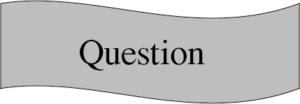## Week 4(Data Analysis)

#### Description:

I wrote a code that reads from the billboard top 100 songs (not the most recent). I edit the .csv file I found online to remove redundant data that I did not need and I used the left data to draw circles at random locations for each of the top 100.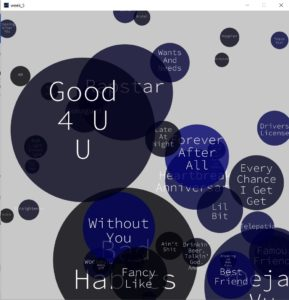I added a small alpha value to show the layering of circles as they are random.

I had to deal with longer song names and make them wrap around.

Instead of trying to find a library that works, I decided to try to make the wrap work myself to explore and know what I can do to manipulate a string.

#### Code:

I started with a circle class to draw each circle at a random location and depending on circle details. I added an array to save the circles.

```String names[];
int ranks[];
int weeks[];
boolean arrDone[] = new boolean;
boolean flag = false;
int counterDone = 0;

PFont myFont;

class Circles {
//attributes
PVector pos ;
int col;
int diam;

String text;

// number of weeks on board gives color shade, and rank determines radius
Circles(int weeks, int ranks, String _text) {
pos = new PVector(random(0, width), random(0, height));
//calculate fraction from 255
col = int((255*weeks/87));
text = _text;
//max diam = 70
diam =int((width)/ranks);
diam = constrain(diam, 50, 500);
}

void draw_circle() {
fill(0, 0, col, 200);
//fill(255,100);
noStroke();
ellipse(pos.x, pos.y, diam, diam);

// split text into lines
String[] words = split(text, ' ' );

writetext(words);
}```

The next function is part of the circle class, I put it here separately because it includes the code for the wrap of the text.

```void writetext(String[] arr) {
String[] temp = new String[arr.length];
int n=0;
for (int i=0; i<arr.length; i++) {
fill(255);
textSize(diam/5);
textAlign(CENTER);
if (arr.length>1) {
if (i<arr.length-1) {
if ((textWidth(arr[i])+textWidth(arr[i+1]))<diam/2) {
temp[i] = arr[i] + " " + arr[i+1];
n++;
//i++;
continue;
} else {
temp[i] = arr[i];
n++;
}
} else {
temp[i] = arr[i];
n++;
}
} else {
temp[i] = arr[i];
n++;
}
}
for (int k=-1; k<n-1; k++) {
if (arr.length>1 && textWidth(temp[k+1])>0) {
if (n>=3) {
text(temp[k+1], pos.x, pos.y+k*diam/5);
} else {
text(temp[k+1], pos.x, pos.y+(k+1)*diam/5);
}
} else {
text(arr, pos.x, pos.y+(k+1)*diam/5);
}
}
}
}```

Here, I declared the circle’s object array and then loaded and processed the data from the .csv file.

```void readData() {
String data[]= new String;
// Convert String into an array of integers using ',' as a delimiter
// string array is returned, which we cast to an int array
names = new String[stuff.length];
ranks = new int[stuff.length];
weeks = new int[stuff.length];

for (int i=1; i<stuff.length; i++) {
data = split(stuff[i], ',' );

//fill arrays with data
ranks[i] = int(data);
names[i] = data;
weeks[i] = int(data);
}
}```

in the creat function here I added a boolean array to check if the song has been output on the screen or not to avoid repitition.

```void create() {
int num =  int(random(1, 99));
boolean flag = false;
if (arrDone[num]) {
flag= true;
} else {
arrDone[num] = true;
}

if (!flag && count<=100) {
// int weeks, int ranks, String _text){
circle[count] = new Circles(weeks[num], ranks[num], names[num]);
circle[count].draw_circle();
count++;
}
}
```

I only used the draw function for the text to appear at the beginning of the code running time. I generated the circles with mouse clicks instead to give time for the user to analyze each new circle.

```void setup() {
size(1000, 1000);
background(200);
}

void draw() {
myFont = createFont("SourceCodePro-Light.ttf", 60);
textFont(myFont);
if (frameCount<25) {
String textt = "Press at a random location";
textSize(60);

text(textt, width/2-(textWidth(textt)/2), height/2-32/2);
}else if(frameCount<30){
background(200);
}

}

void mouseClicked() {
if (frameCount>30){
create();
}
}```

## Week 3 Generative Art

#### Generative Art

This was inspired by one of the things we saw in class. For some reason, I cannot seem to find it, but it was a piece where lines go from a circumference of a circle inwards to fill the circle.

I had a plan in mind to have lines growing out of the center into a circle, but I did not like how it looked, as the lines were too sharp for my preference.

So instead I used the noise function to make random dots, on a 360-degree variation.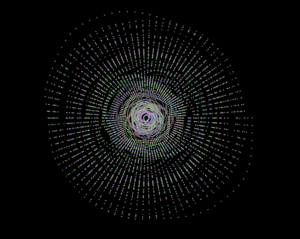I wanted to make it look more natural, however, so I decided to also add some cloudy form around it.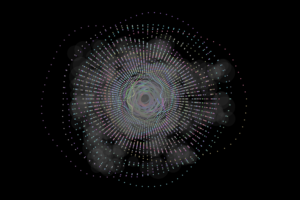When I saw this, I felt like it reminded me of a laptop screen saver, as the circles grow out and it progresses into what it is. Screen savers though, unlike this design above, have different circles it would usually be more than one, so using a random function, I generated the circles starting from a different center point every 100 frameCounts.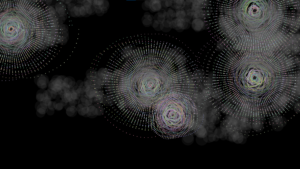That, if I would let go on I would have gotten into a huge mesh after some time, so I called the background function after every 5 circular things are created.

Later I also edited the colors and added an alpha value to make the circles a little easier on the eye.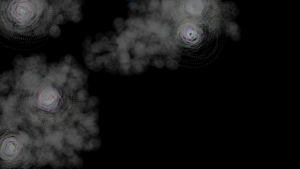and to make it a little user-controlled, I called the background function and reset the center with each click on the screen.

I also decided to make it a full screen like a real screen saver.

#### code:

###### main file:
```Circles _circles;

int centerX = 0;
int centerY = 0;

void setup() {
fullScreen();
background(0);
frameRate(10);
_circles = new Circles(width/2, height/2);
}

void draw() {
_circles.generate();
if (frameCount%20==0){
_circles.cloudy();
}

if (frameCount%100==0){
_circles.setCenter(random(0, width),random(0, height));
}

if (frameCount%500==0){
background(0);
}

}

void mouseClicked(){
background(0);
_circles.setCenter(mouseX, mouseY);
}```

###### Circle file:
```class Circles {

public float xcenter;
public float ycenter;

public float x_pt;
public float y_pt;

public float[][] ptarr = new float;

Circles(float _xcenter, float _ycenter) {
xcenter = _xcenter;
ycenter = _ycenter;
setupp(30, xcenter, ycenter);
}

public void setupp(int num, float x, float y) {
for (int i=0; i<90; i++) {
fill(255, 50);
noStroke();
}
}

//getters and setters
public void setCenter(float _xcenter, float _ycenter) {
xcenter = _xcenter;
ycenter = _ycenter;
setupp(30, xcenter, ycenter);
}

public void generate() {
int offset = 30+(frameCount%100)*5;
float newoffset;
float newoffset2x;
float newoffset2y;
fill(random(100, 200), random(100, 200), random(100, 200), 100);
noStroke();
for (int i=0; i<90; i++) {
newoffset = noise(frameCount + i*0.01)*offset;

x_pt = xcenter + newoffset*newoffset2x;

y_pt = ycenter + newoffset*newoffset2y;

}
}

public void cloudy() {
fill(100, 30);

float new_x = random(xcenter-(frameCount*20)%width/3, xcenter+(frameCount*20)%width/3);
float new_y = random(ycenter-(frameCount*20)%height/3, ycenter+(frameCount*20)%height/3);

for (int i=0; i<99; i++) {
circle(random(new_x-width/10, new_x+width/10), random(new_y-height/10, new_y+height/10), random(40, 80));
}
}
}```

## Simple Work of Art

#### Project Description:

Being creative on a deadline can be a struggle.

I did this design using both circles and “center” mode rects.

In the beginning, I tried using the normal formula for a circle to decide the midpoints of each layer of rect/circles, but for some reason, it did not work as required. So, after a long bugging session, I changed my equations, and I used sin and cos instead.

I started the code with the center circle starting at the top left corner, as it has (0,0) coordinates, making it easier to figure out what I was trying to do.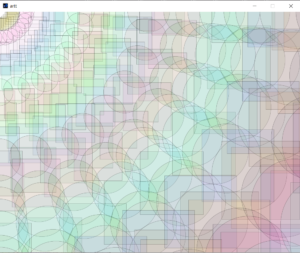After getting this part to work, I made the center point based on the center of the canvas.

I started with the centric shape being all circles with random-fill colors.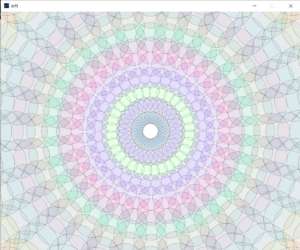But I then thought, why not make a random alternation between both squares and rectangles? It would make it more interesting.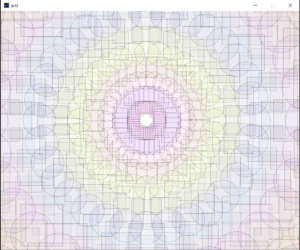I also wanted to make it a little interactive, so I made the center point follow the mouse press as shown in the video.

And that is the end of my project’s story. 🙂

#### Code:

```  import java.lang.Math;

int dimWidth = 1000;
int dimHeight = 800;
int centerX = 500;
int centerY = 400;

void pattern(){
////x=0, y=0;

//println(x);
if(mousePressed){
centerX = mouseX;
centerY = mouseY;
}

for(int j=1; j<50 ; j++){
fill((int)random(0,255),(int)random(0,255),(int)random(0,255), 20);
int num =  (int)random(0,2);
strokeWeight(0.3);
for(int i=0;i<32;i++){
if (num==1){
circle(x_coordinate,y_coordinate,j*10+30);
}
else{
rectMode(CENTER);
rect(x_coordinate,y_coordinate, j*10+30, j*10+30);
}
}
}
}

void setup(){
size(1000,800);
background(255);
}

void draw(){
if (frameCount%10==0){
background(255);
pattern();
}
}```